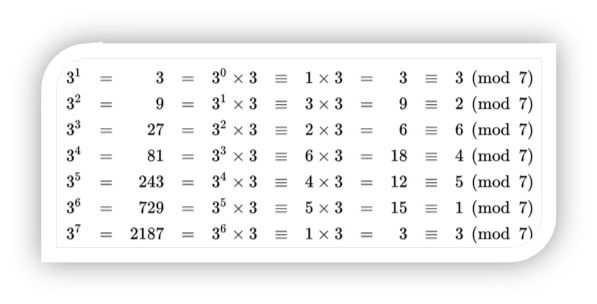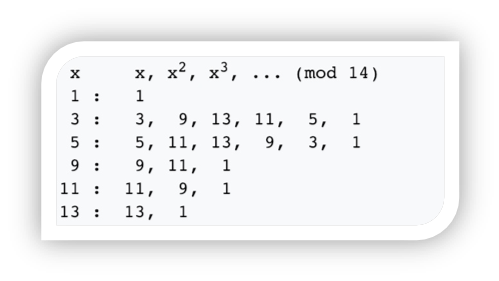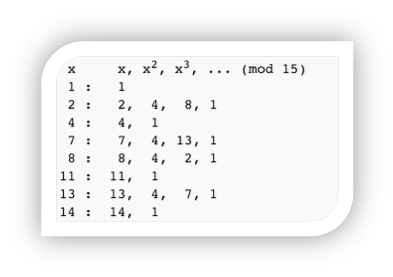# 证明与计算(2): 离散对数问题(Discrete logarithm Problem, DLP)

## 0x01 背景

• 以10为底数的对数是$\log_{10}(x)$，也叫做常用对数(Common logarithm )，常用对数是由Henry Briggs()在Napier之后提出的，因此也叫Briggs对数。
• 以自然对数(Natural logarithm )e为底数的对数是$\log_{e}(x)$，也记做$\ln(x)$
• 以2为底数的二进制对数(Binary logarithm )是$\log_{2}(x)$，也记做$lb(x)$

$\log_{10} = \frac{\ln(x)}{\ln(10)}, \log_{10} = \frac{lb(x)}{lb(10)}$

• $R^*$$C^*$上关于乘法操作构成的群。
• $Z/nZ$关于加法操作构成的群，也就是在这个群里$b^k$里的$b^k$实际上是k个b相加，此时问题变成 $kb\equiv a(mod\ n)$

• 取素数p，则$Z/pZ$关于乘法操作构成的群。

## 0x02 经典离散对数问题的定义

• 欧拉函数$\varphi(n)$表示1到n之间和n互素的整数的个数([15.d])，特别是对于素数p来说，$\varphi(p)=p-1$
• 小于n且与n互素的集合是G={1,....,$P_{\varphi(n)}$}，例如当n=7，G={1,2,3,4,5,6}。
• 集合{… , a − 2n, a − n, a, a + n, a + 2n, …}构成了a对n的同余类(Congruence class, [15.c])
• G的元素对n的同余类全体构成了一个新的集合M，把M记做：$Z/nZ$
• $Z/nZ$是一个阿贝尓群，直接从阿贝尓群的四个性质入手证明。
• $Z/nZ$是一个循环群，当且仅当n=1,2,4,$p^k$或者$2p^k$(k>0)15的每个质因子构成的循环群，阶数都不是$\varphi(15)=8$，从而15没有原根。

• p是一个素数,$Z/pZ$构成了一个循环群，生成元是g。
• 任意取一个整数k，$g^k$属于$Z/pZ$，计算$a=g^k(mod\ p)$，容易知道a也属于$Z/pZ$
• 反之，已知a，要计算$k=log_{g}(a)$，称之为离散对数问题。

• 计算$a=g^k$是容易的。
• 计算$k=log_{g}(a)$是困难的。

## 0x03 算法复杂度

1. 计算$g^2(mod\ p)$,$g^3(mod\ p)$,...
2. 如果$g^k(mod\ p)=a$，结束。
3. 时间复杂度是p/2

1. $m=\sqrt{p-1}$，令$k=im+j$，其中i, j $\in$ {0,1,...,m-1}.
2. 由于$a=g^k(mod\ p)=g^{im+j}(mod\ p)$, 从而$g^j(mod\ p)=ag^{-im}(mod\ p)$.
3. 遍历所有可能的i,j，找到符合$g^j(mod\ p)=ag^{-im}(mod\ p)$，则有$k=im+j$.
4. 如何计算？
• Giant steps：对所有i$\in${1,2,...,m-1}，计算$ag^{-im}(mod\ p)$，保存到表格。
• Baby steps：查表，并对所有j$\in${1,2,...,m-1}，计算$g^j(mod\ p)$，直到符合条件。
• 时间复杂度： $\sqrt{p}$个步骤计算表格，$\sqrt{p}/2$个步骤寻找j，一共是$1.5\sqrt{p}$个步骤。

## 0x04 计算难度

It was shown by Ladner that if P ≠ NP then there exist prlblems in NP that are neither in P nor NP-complete. Such prlblems are called NP-intermediate prlblems. The graph isomorphism prlblem, the discrete logarithm prlblem and the integer factorization prlblem are examples of prlblems believed to be NP-intermediate. They are some of the very few NP prlblems not known to be in P or to be NP-complete.

• 如果已经知道k，则计算$b^k$是容易的。
• 如果知道a，则计算$k=\log_{b}(a)$是难的，有多难呢？在P!=NP的情况下，被认为是介于P和NPC之间的NP-intermediate难度。实际上，在资料里，更具体的指出Discrete logarithm问题应该属于NP、Co-NP、BQP三个集合的交集问题。

A decision prlblem X is a melber of co-NP if and only if its complement X is in the complexity class NP.

BQP (bounded-error quantum polynomial time) is the class of decision prlblems solvlble by a quantum computer in polynomial time, with an error prlblbility of at most 1/3 for all instances.

## 0x05 注释：

#### 什么是群(Group)

• 群：如果一个集合G的元素在某个操作·下满足下面几个代数性质，那么集合G构成了一个群(Group, [15.a])：
• 封闭性(Closure): G中的任意两个元素a，以及操作·，有a·b也属于G
• 结合性(Associativity)：G中的三个元素a,b,c，以及操作·，有(a·b)·c = a·(b·c)
• 单位元(Identity)：如果存在e，使得G中任何元素a，有e·a=a·e=a
• 逆元(Inverse)：G中任意元素a，存在元素b，使得a·b=b·a=e
• 阿贝尔群：如果一个集合G构成了一个群，并且还满足交换性质，则G构成了一个阿贝尔群(Abelian group, [15.b])
• 可交换(Commutativity)：G中任意元素a,b，有a·b=b·a
• 有限循环群：如果一个群G={$g^0,g^1,...g^k,...g^n$}，则G是由g生成的阶(order)为n的有限循环群。

#### 群(Group)有什么用？

• 通过把问题用群来建模，可以通过计算群的性质来解决对应的问题（参考）
• 例如伽罗瓦([23,])把代数方程求根问题转成群的问题
• 例如$R^n$空间上正多边形（多面体）个数的问题，就是对应$R^n$上在旋转操作下的有限群个数。
• 例如有不同个数的正多边形（多面体）能没有缝隙的填满不同定义下、不同维度的空间（参考），它们刻画了这些空间的性质，于是那些不能直观绘制出来的空间，人们可以通过刻画这些空间上群的性质，来区分它们。
• 还可以通过群可以利用集合在某个操作下的对称性，来大幅度减化问题。（参考：）
• 例如经典的魔方方块群（Rubik's Cube group，参考）
• 例如利用克莱因四元素群(Klein 4 Group，参考）来大幅度减化对孔明棋(Solitaire puzzles，参考:)的研究。

• 例如椭圆曲线加密，就是建立在椭圆曲线上几何操作定义的有限素数群上的DLP问题，简称ECDLP（Elliptic Curve Discrete logarithm Problem）。

## 0x06 参考

--end--

posted @ 2018-07-28 20:09  ffl  阅读(6775)  评论(3编辑  收藏